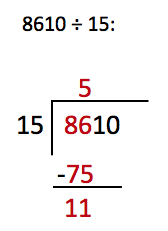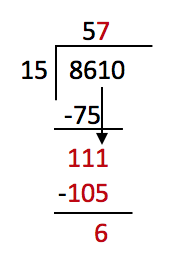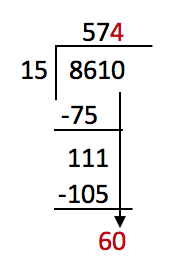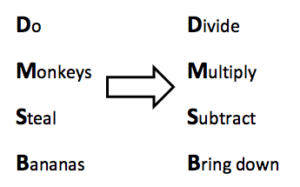What is long division? Long division is a method of dividing larger numbers (3 or more digits) by numbers of 2 or more digits.

This is how to set out long division:First, 15 does not go into 8, so look at the next digit.
15 goes into 86 five times, so write the number 5 above the 6.
(15 x 5 = 75)
Next, to work out the remainder take the 75 away from 86.
(86 – 75 = 11)Then carry down the 1 to make 111.
15 goes into 111 seven times, so put a 7 above the 1.
(15 x 7 = 105)
Now take 105 away from 111 to get the remainder:
111 – 105 = 6Finally, carry the 0 down to make 60.

15 goes into 60 exactly 4 times, so put a 4 above the 0.
(15 x 4 = 60)
This gives you the answer 574

(8610 ÷ 15).

## When do children learn to do long division?

At the start of Key Stage 1 children will be taught the concept of division, teachers will probably introduce this by getting children to share out some objects amongst themselves. For example, some children might be given 6 coloured blocks and then asked to give half of them to the classmate sat next to them.

In Key Stage 2, after learning all their times tables and division facts, children will begin to use short division (called the ‘bus stop’ method) in Year 5. Short division is used to divide 3 or 4 digit numbers by a 1 digit number. Teachers will then introduce children to the long division method above so that they can use it to divide larger numbers by 2 digit numbers.

## How to help children with long division?

Using the long division method will require that children are confident in their times tables and that they understand how multiplication relates to division, as there are a lot of calculations to work out as they go along. Therefore, if children are struggling it could be a good idea to go back over their times tables and make sure that they know their division facts. For example,

if 6 x 4 =24, then 24 ÷ 6 = 4.

It is also important that children understand the different terminology used in methods like long division. You may need to explain that a remainder is the number left over from a calculation. For example:

The number 27 does not divide exactly into 5, but we can divide 25 exactly by 5 (as 5 x 5 = 25). So, if Harriet had 27 sweets to share between her 5 friends, each friend would get 5 sweets and Harriet would have 2 sweets left over.

To remember which order to do calculations in long division, it can be helpful to create an acronym to make it more memorable. For example:## How does Learning Street help children with long division?

Similar to what they will be doing at school, Learning Street will begin with the basics of division in earlier courses, as without knowing the fundamentals, the child can’t learn long division. Through extension and revision, the child will slowly develop their knowledge of division before then being introduced to long division, followed up with extension and revision.

Tests could include SATs, competitive 11 Plus tests or selective Independent school exams.

## Our Courses

Click through to review the courses we have available

##### Get Started

Learning Street structured courses make home study easier and more successful. There's no need to wonder which books to buy or what to do next. Everything is planned for you.

• Proven to deliver excellent results
• Used by families, private tutors and schools
• Fully planned and structured